## Wednesday, 29 December 2010

### The Poisson Variation of the Binomial

My recent post about Why the Other Line Moves Faster reminded me of another variation of the binomial distribution that is not covered in AP Statistics, the Poisson Distributions. (Ok, the Poisson is lots more than just a special case of the binomial, more about that later, but in the days before hand-held calculators it was in that sense that we met it.)

The distribution is named for the French Mathematician Simeon Denis Poisson (hey, his name is on the Eiffel Tower.. 2nd from the right on the South East side).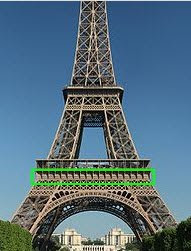Suppose you had a very rare binomial event, let's say something that happened only one in 200 trials (p=.005) and we wanted to know how probable it was to happen four times (x=4) in three-hundred trials (n=300). The calculation of the binomial probability is now as easy to do with modern calculators as with the Poisson approximation to it, but in my youth a calculation of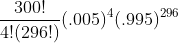looked nearly impossible.

The Poisson frequently uses the Greek letter lambda,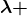, for the mean or expected value np. In this case NP=300(.005) = 3/2 indicates that, on average, we would expect only 1.5 successes in 300 trials. We want to calculate the probability of getting four successes. The Poisson probability is given by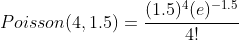. The difference between the two calculations is less than .0002 on my Ti-84 calculator.

The Poisson is not limited to binomial events. More often it is applied to events which are distributed randomly across time. The same calculation above could be used to calculate the probability of four people entering a bank in a 15 minute period when only and average of 1.5 visitors would be expected. If a manufacturing process produces an average of 1.5 failures per day and you wanted to calculate the probability of four failures, you use the same calculation again.

And if you wanted to know how many cashiers to keep open at the market, how many mutations in a string of DNA exposed to radiation, the number of deaths from a rare side effect of a drug, or other similar situations which involve a very rare event with a very large number of possible incidences, the Poisson will often suffice as long as conditions of independence are met.

The Poisson distribution is sometimes called the Law of Small Numbers after a book by the same name by Ladislaus Bortkiewicz published in 1898 on the Poisson distribution.

For AP Statistics Students, it is often possible to approximate the Poisson with the Normal Distribution as long as the value of lambda is about ten or more. For the Poisson, the mean and variance are both the same, so if you approximate it with a normal, use a mean of lambda, and a standard deviation that is the square root of lambda. Do remember to do the continuity correction for the fact that the Poisson is discrete and the Normal is continuous.

### Why the Other Line is More Likely to Move Faster

One of my ex-students, Chad Brinkman, sent me a link to this video by Bill Hammack, "The Engineer Guy,". A nice view for any math student.

Video: Why the other line is more likely to move faster## Friday, 24 December 2010

### A Happy Holiday to All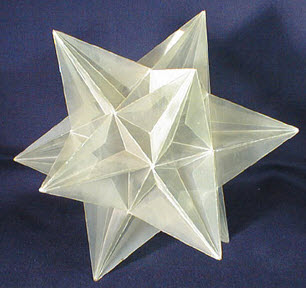Until the world finds the peace we dream of... May you find peace in your own heart.

And a carol I snitched from John D. Cook at his "Endeavour" Blog

## Thursday, 23 December 2010

### More "Almost Binomial" Distributions

In my recent post I illustrated the extension of the binomial to a Multinomial Distribution. In a similar way, the geometric distribution and the Pascal (aka the Negative Binomial) Distribution are very much like special cases of the binomial.

I will illustrate each with a simple probability example. In a limited version of the game of "greedy pig" you roll a die as many times as you wish each turn and you add the points on the top of the die to your score for that turn...but... if you roll a one, your turn ends and you lose all the points you have earned for that round. One might inquire, what is the probability that you could roll the die n times without rolling a one. Since the probability on each roll is the same, we could handle this using the binomial (or multinomial) distribution with n trials, p=5/6, and the number of successes also equal to n. For n=5 for example, we get (using the notation established in that blog)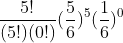which simplifies to just (5/6)5 .

But a slightly different question might be, what is the probability that our first failure (rolling a one) would occur on the sixth roll. This is asking for the probability that the first five rolls succeed, and then the final roll is a failure. This is the general model for a geometric distribution. The reason it is called a geometric distribution is clear if you calculate the probability of the first failure happening on the first, second, etc rolls.

Roll...1....2.......3........4...

Prob...1/6..5/36...25/216 ..5^3/6^4..

notice that each probability is the previous probability multiplied by a constant ratio of 5/6. The terms for a geometric sequence (which must sum to one to be a probability distribution......check)

In general, if the probability of failure is q = 1-p.. then the probability of the first failure occurring on the nth trial is given by (p)n-1(q)

It often surprises students that the mean for such a distribution is 1/q where q is the probability of a failure. OK before I confuse someone.. the geometric distribution is sometimes described as the number of trials to the first success, so you may see the expected or mean value as 1/p. In any event, if the probability of an event happening (whether you call it success or failure) is p, the expected number of trials before it happens is 1/p.

Now if you are really clever you can figure out how to do the next problem without me, but let's walk through it anyway, (hey...it's MY blog).
Suppose instead, you could keep rolling until you had three rolls of one..... sort of "three strikes and you're out." Now what is the probability that the third strike comes on the tenth roll.
The idea of course, a collection of 9 rolls with 2 failures anywhere in the string, and then a third failure on the tenth roll. To get the probability of all the possible ways to get 7 successes and 2 failures in the first nine rolls is a straight binomial (multinomial) probability problem.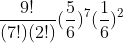We just multiply this by a failure on the tenth roll and we have the probability we seek. Since we have a couple of "failures" in that (1/6)^2, we might as well just up it to a three and be done. The final probability is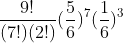If you would like to experiment with these distributions, I came across a nice experimental applet hereThis experiment uses the trials to k successes instead of failures, and so p and q are switched here (and it seems I could only adjust these in .05 increments). This is a nice routine and you can simulate trials by clicking on the "step" button to see how many trials it took to get three successes.
This applet is part of a nice virtual laboratory created by Kyle Siegrist of the Department of Mathematical Sciences at the University of Alabama in Huntsville. There is lots of nice stuff. See the home page here.

When we deal with integer numbers of failures this is called a Pascal Distribution after Blaise Pascal. It can be extended to any real and is then called a Polya Distribution, after George Polya. This has application for events which are very rare, but related to each other, such as hurricanes. Both are special cases of the general Negative Binomial Distribution.

## Wednesday, 22 December 2010

### Extending the Binomial Distribution

Almost every high school student is exposed to the binomial distribution in some form. They may see it in expanding binomials such as (x+y)4, and they may also come across it as a method of solving simple probability problems... "what is the probability that a family with four children will have three boys and one girl?"

The ability to naturally extend the binomial (or to recognize that the two questions above are interrelated) is probably hampered by the notation used in the "choose" command, or the various combination notations. The normal way for students to address the problems about combinations is to think of one group embedded in the total field. They may useto find three boys, or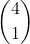to find one girl. Both methods lead to the same calculation,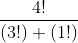but they seem to direct the focus of the learner away from the idea of "three of these and one of those" which would embed the problem firmly in the multinomial distribution. I suspect that if the "choose" or "combination" notation was not used, many students would almost naturally extend the binomial probability problem to similar problems with three (or more) item choices.

For students who have never seen the multinomial I will provide a brief introduction, and a few good links.

Suppose instead of two choices to pick from, a population had three choices..(the extension to four should jump out at you). A spinner has the numbers one, two, and three on it with probabilities of 1/6, 1/3, and 1/2 respectively. What is the probability that in ten spins you would get 2 ones, 3 twos, and five threes. The probability is simply given by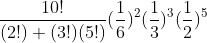The association between the number of things selected in all (10) and the number and probability of the individual partition seems to be naturally extendable to any number of items. Keep in mind that, like the binomial, this requires that the probability on each draw is unchanged... we are drawing with replacement or from an "infinite" pool. This does require that the sum of all the probabities add up to one

You can use this to extend the expansion of a binomial to the expansion of any polynomial to a power. To make this clear to new learners, I will go back to the idea that (x+y)4 is related the probability of three boys and one girl in a family of four children. To do that, I want to give a verbal expansion of (x+y)4, but instead of x and y I will use b and g for (boys probability of birth and girls probability of birth... well, they might not be 1/2). The expansion of (b+g)4 will give the probability of every possible outcome, 4 boys and no girls, 3 boys and one girl, two boys and two girls, one boy and three girls, and no boys and four girls. Each term in the expansion represents one of these cases. For four boys and no girls, we have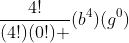, this is added to each succeeding term until we end with no boys and four girls. This gives exactly the expansion you would have for (x+y)4 except for the use of b and g as variables.

To extend this to a trinomial we get a few more terms, but we can just attack them systematically as there is no such natural approach as there is in the binomial case. For instance, if we had (a+b+c)2 we could have two a's, two b's, two c's, or ab, ac, or bc so there must be six terms. It may help to think of it as (a+b+c)(a+b+c) and you pick one term from the first trinomial and one from the second to multiply. The three squared terms will have coefficients of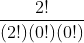which is a big one. The ones where we pick two different ones will be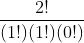, which can occur two ways. So we get a2+b2+c2+2ab+2ac+2bc.

If you want to try (a+b+c)3 then you will get ten terms. In fact for any power n, a trinomial will have the n+1st triangular number which coincidentally is (n+1) choose 2. There is even an extension of Pascal's triangle, called Pascal's tetrahedron that can be used but you have to create it (or at least I do) level by level. I find it usually easier to just do the multinomial coefficients. You can find a pretty good explanation of the tetrahedron here. There is also a good wikipedia page about the multinomial distribution.

## Tuesday, 21 December 2010

### Cool Doodles

One of my favorite ex-students sent me a link to this video by Vi Hart. I loved it, but I hope Jessica didn't find my math class as awful as Vi describes them. Anyway, enjoy.

Vi has her own web page with lots of math and music stuff.

## Saturday, 18 December 2010

### "Decimal" Fractions in Other Bases

Early in my study of decimal fractions I realized that the ninths were just repeating digits of their numerators. 1/9 = .111...; 5/9 = .5555... etc. I didn't have much to apply it to, but it sort of fascinated me. Somewhere in the sixth grade or so, we were introduced to bases other than ten. Something about Sputnik made American education decide that base two and base five was important. I was fascinated again, but when I became curious about "decimal" fractions in other bases, my teacher advised me that, "We don't cover that." {If you know a good name for the general term of such fractional expansions, please advise.} Thanks to GasStationWithoutPumps, I now know this is called a radix expansion. Radix is from the same base that gives us "root".

Later I began to understand polynomials better, and realized that I could extend base n whole numbers across the "decimal" point as far as I wanted using the idea I would now describe as negative powers (not sure I had a word for it then). Armed with the idea that .1 in base 2, or .1  was 1/2, and .1 in base 5, .1 was 1/5 I began trying to construct sets of fractions. Moving the "decimal point" one to the left in base two divided the result by two in the same way that it divided by ten in base ten. With that, I could produce most of the fractions that terminated, .0101 was 1/4 plus 1/16 or 5/16, .23 was 13/25 (2*5+3)/52.

Then I read about the formula for infinite geometric series with ratios less than one. I think the article was about Archimedes use of the series, but I couldn't understand the center of gravity approach at that time. What I did realize was that I could use the formula to convert any non-terminating decimal fraction to a rational fraction..... King of the World. I would write out strange fractions that had non-repeating prefixes to the repeat. Soon I began to wonder about repeating decimals in other bases and set out to explore. Remembering the repunit expansion for 1/9, I wondered about .1111... in other bases. I was kind of shocked to realize that .11111.. was =1. How could that happen? But I had already read about "proofs" that .99999.. = 1; and quickly convinced myself that in base n, a repetend of the digit (n-1) would also be one. But somewhere along the way, I realized that .1111...[n] would be 1/n-1. Just as it was equal to 1/9 in base ten, it was 1/4 in base five, or 1/2 in base three.

With all this experience, I still found it very hard to pick a random fraction, say 4/7, and express it in base 3 or base 5 or whatever I wished. Then one day I learned about division. Ok, I had learned long division and short division and mental division tricks, but I didn't really know how division worked. I'm not sure what I was reading, thumbing through books in the public library, and the author showed a shortcut for making "decimal" fractions in base two. What seemed like a magic trick became understanding when I began to extend it to other bases.

To understand, I want to do a simple division in base ten written a little differently than you normally would. For an example I will use 1/8. Set up the operation in four columnsSince 8 will not divide into 1, we have a fractional answer and we will multiply one by ten and try again (this is actually dividing the number of tenths by eight. This time 8 will go into ten once, with a remainder of two. This is shown in the second line.The remainder is 2 multiplied by 10 (to get the 20) and we divide by eight again. This continues until we either terminate, or enter a repeating pattern. Here is the final table giving us the expected .125 for an answer.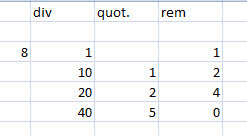The question is what is special about ten, and the big answer is ..... nothing. We could divide the fraction in any base by simply using some other multiple in place of the ten in each line. Here is 1/3 in base five.Notice that the occurrence of the remainder of one means we will repeat the same sequence forever, so our answer is .13131313...  = 1/3. We can convince ourselves this is correct by using the geometric series. The first two digits are 1(5)+3=8 and represent 8/25. The next repetition of 13 is 8/252 , and each two digits in the sequence is 1/25 of the previous two. This is a geometric sequence with a first term of 8/25, and a common ratio of 1/25. Using the well known formula for such series gives 8/24 = 1/3..

Ok, one more example of the division method to help you.... this time we pick base three, and let's try to represent 2/5 in that base.2/5 = .101210121012..... OK, one more quick tip. Most students know that any repeating decimal fraction can be written as a rational by just subtracting one from the denominator of the repetend (say WHAT?) ok.. .4 repeating is 4/9 (four tenths repeating); and .232323... is 23/99. It doesn't matter how long the repeat cycle is, as long as it starts right from the decimal point; .12345 is just 12345/99999..... and you can do that in ANY base...
so a fraction like .1012 in base three can be written as its base three fraction and then apply the same rule. 1012 in base three is 1(27)+0(9) + 1(3)+2(1) so the numerator is 32 in base ten, and the denominator is 34 or 81. The rule for repeating is subtract one from the denominator, so .10121012... is 32/80 = 2/5....

.101 repeating in  = 5/(23-1)= 5/7
.101 repeating in  = 10/(33-1)= 10/26= 5/13
.31 repeating in = (3(4)+1)/(42-1)= 13/15
.31 repeating in = 16/(52-1)= 16/24 = 2/3
.31 repeating in = 19/(62-1)= 19/35

Fun with fractions!!!

## Wednesday, 15 December 2010

### Viete on Combining Pythagorean Triples

Not able to write much this holiday season, but here is one I really enjoy from a few years ago.. hope it is of interest...

Reading The Analytic Art by Francois Viete, or at least the T R Witmer
translation, and came across an interesting way of combining the legs of any two Pythagorean triples to create two others. Viete calls the two methods synaeresis and diaeresis, which seem to be language terms Viete appropriated. Synaeresis is cramming two vowel sounds together to make one... like the way people in New Orleans say "Nor"leans. I think the official term is diphthong, but check with an English major for confirmation. The actual Greek roots mean “a joining or bringing together" or something similar Diaeresis is stretching one vowel out into two....and you can find your own example...
To illustrate Viete's approach, we can take two simple right triangles, say a 3-4-5 and a 5-12-13 as examples. Viete's method would produce two triangles whose hypotenuses( hypotenii?) were both 5x13 = 65 units. Viete distinguished between the legs calling them base and the perpendicular, so in the 3-4-5 triangle the base is 3 and the perpendicular is 4. It doesn't matter which is called what name, of course except that it reverses the outcomes of the two methods. The Synaeresic method would be to add the products of each base with the perpendicular of the other triangle; 3x12+ 4x5 = 56. This would give one leg of the new triangle. To find the other leg take the difference of the products of the two bases from the two perpendiculars; 4x12 - 3x5 = 33. This completes a triple of 33-56-65.
The second method, simply reverses the signs of conjunction. Subtract the two perpendicular x base products and add the two products of a common part. The crossed terms gives 3x12-4x5 = 16 for one leg, while the products of like parts gives 4x12+3x5=63 for the other, completing a 16-63-65 right triangle.

## Friday, 19 November 2010

### Repeat of an Isoperimetric Discovery

It's that time of year again, so I want to repeat this to explain some ideas I laid out in Calculus today...

Recently we have been doing maxima/minima applications in calculus. By the time they get her most of them know that when they maximize the area of a rectangle with perimeter constraints, they get a square. In Pre-calc we do some basic problems with 2 adjacent pens, a single pen against a wall or river, and all the similar cases. I have written about the generalizations of some of these here, and here. The problem of two adjacent congruent pens is (or should be) easy for a calculus or precalc student.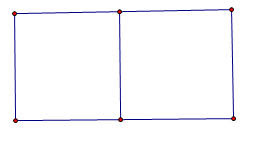It turns out that the maximum occurs when the total width is one-fourth the perimeter and the height is one-sixth the perimeter, for a maximum area of one twenty-fourth of the perimeter value (in square units, of course). If you extend that to even more congruent rectangular pens placed end to end it turns out that the width stays the same?

If you extend this, as many teachers do, to a shape like this one, it starts to get interesting to the more observant student.The height is now one-eighth of the total , and the width is one-sixth of the perimeter. Even slow students can figure out that the area of the total turns out to be one forty-eighth of the value of the perimeter. Some will even generalize the area of an array of mxn congruent pens to be the perimeter squared divided by [4(m+1)(n+1))].... but it seems to take forever for some one to finally realize that in all these problems, whether there was one rectangle, or one with one side adjacent to a river or barn, or rows of rectangles... there is one invariant...

Theorem one for rectangular arrays of congruent rectangular pens "The amount of fence going right to left is the same as the amount going up and down." If you want to maximize a rectangular array with five rows of three pens in each row, we will need 24 lines of fence, four going vertically, six going horizontally. Use half the fencing to make the four vertical fences, and the other half to make the other six going horizontally. (Prove..... dear reader) ..

I always thought this fifty-fifty use of fencing was a cute mathematical "teacher trick" but I have recently come to believe there is something deeper at work. I played around recently with this problem but loosened the constraints on the pens to be just equal area rectangles rather than congruent. This offered some nice areas to explore. Which would be better, three congruent pens in a row, or two pens with a third beneath... like so.The three adjacent pens turn out to have a max area when the width was p/4 (where p is the perimeter) and the height was p/8, giving a maximum area of p2/32... and as before, that mean that half the perimeter was used for the vertical sections, and half for the horizontal sections..and for the three non-congruent sections with equal area the width was p/6, and the length was 3P/16, giving a max area of....wait, that's also p2/32. (Ok, I hadn't expected that)... but when I checked the dimensions, it turns out that the horizontal sections use up exactly half the perimeter, and the vertical sections the other half in both cases.... hmmm...

Ok, but every kid knows that the area is maximized when the rectangle is closest to a square... so what if we just put three squares arranged as shown below and didn't require that the whole assembly forms a rectangle... I thought that would probably be even more area..It turns out that it isn't, The equal distribution of left and right still works out, but the total area is only 3p2/100 or about 96% of the other shapes. (ok, I wasn't ready for that)...

So, I decided to move up to five pens... I passed over four absolutely sure that the division into four equal squares in a two by two array would be the most efficient, but five offered several variations, five in a row; two rows of two with a single in the third row, or three in one row and two in another... The five congruent squares in a row gave a max area of p2/48, but the set up with three pens in one row and two in another gave a slightly larger area of 5p2/216 or p2/43.2. The 2,2,1 set up (shown below) gave not quite the same area..This set up gave a max area of p2/44.8.

From all of this I have a formed a totally unproven conjecture about the maximum area when n equal area rectangular pens are set out in a rectangular boundary with a given amount of fencing.
The Theorem One above for congruent rectangles seems to be a stronger property than I suspected, and is applicable to all equal area pens in a rectangular boundary, And the conjecture is that when the number of pens, n, is between x2-x and x2+x, the maximum area will be found when there are x-rows of pens and all of the pens have either x-1, x, or x+1 pens in the row.

The number of pens in any row will never differ by more than one from any other row. For values of n that are perfect squares or pronic (product of consecutive integers), the number of pens in each row will be the same. When n > x2 there will n-x2 rows that have x+1 pens, and the remaining rows will all have x pens. Similarly, if n is less than x2 there will be x2 - n rows that have x-1 pens and the remaining rows will have x pens each. As an example, if n= 23, then x=5 since 52-5 is less than 23 which is less than 52+5. And for 23 pens there will be five rows with x=five pens in three of them, and x-1=four pens in two of them.

I also have discovered that the theorem about equal lengths of fencing horizontally and vertically seems to be more important as a "rule of thumb" for maximizing than is equal sides. For example if five squares are set out as efficiently as possible (four in a square with one attached to a side), the area will be p2 / 45 with 8/15 of the perimeter going one way(say horizontally) and 7/15 going the other. If we change the squares to rectangles with each having a height of p/16 and a width of p/14 the area is p2/44.8.. an improvement of about 1/2 %....
Even if you go to six pens (where there is no lost perimeter enclosing the odd pen), putting two rows of three squares gave me an area of P2 / (48 1/3) but using the equal division of fencing gives the very slightly improved maximum of p2/48.

I can't explain why, but the equal division of fencing theorem is stronger than I first suspected, and if someone out there can shed more light on it than my feeble exploration, please do.

I haven't had time to expand the formula for the max area of all n when a rectangle is divided into congruent area rectangles, (not too difficult, I think).. but for square or pronic numbers of pens, it seems the max area will always be p2/(4 (x+1)(y+1)) where xy=n. As a limit as n approaches infinity, it seems the max area for rectangles divided into n equal area rectangles approaches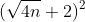## Thursday, 18 November 2010

### Plus or Minus InfinityCame across the problem above at the Math Central web page from Canada. I had written before about the values of iterated roots, but had not considered the case of what happens if they are joined by subtracting instead of adding. It turns out that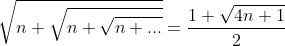. The derivation of this can be found in a short paper I wrote here.

By a similar approach we see that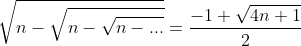making the problem above pretty simple as an aftermath of all this... but I wondered if there was an intuitive approach to justify this without the actual itereation results....nothing jumps out yet....

It also made me wonder about alternating signs...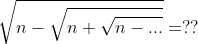.

Playing around with this I came up with a fourth degree equation to solve, with two positive roots...Yikes, but one of them was a spurious solution created by a second squaring of the equation. When I worked it out for n=2, I got a real surprise.. it led me back to the itereated sequence which started me on this exploration way back when...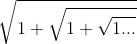... it turns out that the iteration of roots of two using alternating signs is deeply related to the iteration of roots of one using addition. I'll leave it to you to explore...

And their difference, if I have calculated correctly, is the iterated square roots of 3 using alternating signs.... Too much to explore,...... more later, but I just figured out in class today (I hope) that the series with alternating signs will equal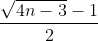and produces an integer value when the value of n is =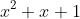for integer x.

## Wednesday, 10 November 2010

### Centroids of Inscribable Polygons

The term centroid, or center of gravity, of a triangle usually refers to the center of gravity obtained by treating the vertices as point masses with a mass of one unit. This leads to the centroid noramally found in geometry by intersecting the three medians. If we have points (xA,yA), (xB,yB), and (xC,yC) it is well known, and easily derived, that the centroid would be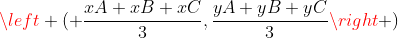.

There are other types of centroids, and one of them has an interesting relation to the class of polygons mentioned in the title. Another center of gravity can be found if we use the boundary of the polygon and assume it is made up of uniform rods. For a triangle, this produces a center of gravity that is at the intersection of the angle bisectors of the medial triangle (the triangle with vertices at the midpoints of the original triangle). This point is also called the Spieker Center of the triangle. To distinguish these two types of centroids, I will call the center of gravity of the vertices Gp (for point) and the center of gravity of the boundary Gb.

Some interesting sidebars about the angle bisectors of the medial triangle before I continue about centers of gravity.... The angle bisectors of the medial triangle are always parallel to the angle bisector of the opposite vertex. Also, these lines are each a "cleaver" of the triangle; a term created by Dov Avishalom in a Mathematics Magazine article for a line that passes through the midpoint of one side and divides the perimeter of the triangle into two equal halves.

I recently came across a paper by Tom Apostle and Mamikon A. Mnatsakanian that pointed out that in any triangle the two centroids and the incenter were always collinear. They also show that the ratio of the distances of the two centroids from the incenter is always in a ratio of 3/2. The ratio Gb/ Gp= 3/2.

Then in a really nice generalization, they showed that the same property applies to any inscribable polygon, one in which each edge is tangent to a common circle, such as the quadrilateral below. They use the clever term "circumgons" in the paper for such shapes. This is really a nice term since "inscribable" and "circumscribable" are used both to describe the circle inscribed in a polygon, or the polygon circumscribed about the circle... even I get confused.. Since it is often much easier to find Gp and the incenter of inscribable polygons, this provides a clever way to find Gb.

Students may be interested to know that Archimedes used the formula Area= 1/2 P r for the area of a circle, where P is the perimeter (circumference). This is, of course, simply and alternate form of the formula they learned in grade school. They may be even more surprised to find that the Archmedian formula gives the area of any inscribable polygon if P is the perimeter of the polygon, and r is the radius of the inscribed circle. The proof is very easy, and is often provided in high school textbooks for the limited case of regular polygons which would always be inscribable. The image below provides non-subtle clues to the proof.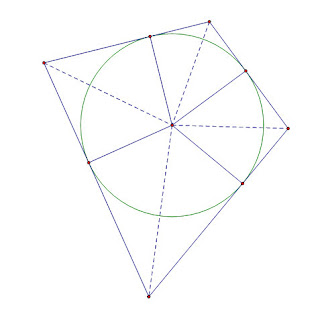## Friday, 5 November 2010

### Dollar Bill TrigonometryJust in time for my introduction to trig identities, Kate Nowak at f(t) reminded me of a beautiful geometric property of dollar bills.

Kate shows how to fold the dollar step by step, transforming it along the way from a rectangle to an isosceles trapezoid, then to a rhombus, and finally an equilateral triangle. And you can tease out a pretty decent regular tetrahedron with no overlap.

All this goes to show that the ratio of the height and width of a dollar bill is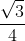...
But that is an important number.. that is the area of an equilateral triangle with unit sides. So if you made an equilateral triangle with all three sides the width of a dollar bill, then it would have the same area as the dollar bill... And the tetrahedron made from the dollar fold has the same surface area as well.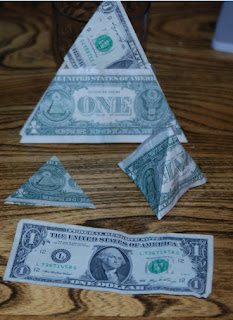The first two folds are pretty interesting for even a more advanced class... they form a 30-60-90 triangle, and it might be kind of nice to challenge students to explain WHY??? If it is not a 30 degree right triangle, then the whole equilateral triangle thing is a mess. The key is actually in recognizing that we have done what students/teachers may have heard is impossible, we have trisected an angle.

I was first introduced to this little dollar folding trick by a fourteen year old in my algebra II class some years ago..."Hey, Mr Ballew, bet you can't fold a dollar into an equilateral triangle." After I figured it out... (it took me a while).. I challenged him to explain why the first fold made a 30 degree right triangle. I can't tell you how proud it made me when seconds later he said, "Oh, it's because all three angles are equal."Quite clearly the folded portion is the same angle as the triangle where it would be "unfolded", so those two angles are congruent...and the third angle, well the length of the hypotenuse is one dollar bill height, and the opposite side is 1/2 a dollar bill height, so it is a 30 degree angle...which means the other two congruent angles must each be 30 degrees...

SPOOKY fun with folding money

Ok, it works with a fifty or a hundred bill too, all US bills are the same dimension (not true in most countries)...but hey, I'm on a teacher's pay, so I use ones...

Truth in Blogging Details.... AS wonderful as I would like this to be..the dollar seems NOT to be in a ratio ofexactly... Most places I have searched say the US dollar bill is 6.6294cm (2.61") wide, by 15.5956cm (6.14") long.. that is a ratio of 0.425081433... the square root of three divided by four turns out to be about 0.433012702... a difference between the two ratios of .008....

## Thursday, 4 November 2010

### Complex and Other Conjugates

We had covered the Fundamental Theorem of Algebra in pre-calc and after a few days of trying to make sure they understood how to decide how many real and non-real solutions there were to a real valued polynomials and that they could be factored over linear and quadratic factors , I was trying to get them to see how easy it was to work back and forth from the quadratic polynomial and the complex zeros. Finally I told them, "It's almost like the Pythagorian Theorem. a2+ b2 = c " [They will later learn that this is really just the product of two equal pythagorean results for each of the conjugates, but not yet]

I showed them that the complex solutions always came in conjugate pairs, a + bi and a - bi... and that if they knew the zeros, they could create a quadratic which had those solutions. It seems that students are no longer exposed to what I call the sum and product property of quadratic zeros... that if the zeros of a quadratic are r and s, a quadratic with those zeros is given by x2 - (r+s)x + (rs)... (If you knew the zeros were at x=3 and x= -1, then a quadratic with those zeros would be x2 - (3-1)x + (3*-1) or x2 - (2)x -3).

They had to get it somewhere if they were ever taught to factor, but it never seems to find purchase in their mathematical memory. I pointed out that for complex conjugates, r+s was real since the complex +bi and - bi eliminated each other, and so r+s was just twice the real coefficient of either conjugate. I connected this to the graph by showing that this "a" in the a+bi was the axis of symmetry of the graph. For example a quadratic with complex zeros at 3+2i and 3-2i will have a linear term of -(3+3)x [assuming we use the easiest case with A=1]. This also means that the of the quadratic will have its vertex at a point with an x value of 3.

It is also easy to see the constant term of the quadratic. If you multiply (a+bi)(a-bi) the distribution gives a2 +abi - abi - b2i2... As with the sum, the two imaginary terms will cancel out, and since i2=-1, we see that the product of the two complex conjugates will always be a2 + b2. So for the example 3+2i and 3-2i, the product of the conjugates is just 9+4=13. Putting this in as the constant term, we see that the quadratic x2 - 6x + 13 has zeros at 3+2i and 3-2i.

This gives another way to solve a quadratic equation with complex roots. If we have a function x2 - B x + C that we know has complex solutions, we know that the real coefficient, a, of the conjugate pair will be -B/2 (shades of the quadratic formula)... and we know that a2 + b2 = C, so we can figure out b pretty simply. If we had a quadratic such as x2 - 8 x + 25 we would quickly see that the real coefficient is -(-8)/2 = 4... and know that 42 + b2 = 25, so b must be 3, and the two solutions are at 4 +/- 3i. I finally admit to the students that this is just a variant of the quadratic formula, and sooner or later someone will ask if it is not possible to do the same thing with a quadratic with real zeros... I let them suggest a quadratic, and only press for the use of an even B in the linear coefficient for ease of explanation. For example with x2 - 6 x + 5 (ok, I picked an easy one).. we would see that the "a" part was three again... but now we have 32 + b^2 = 5.

If we remember that b was the imaginary coefficient, it makes sense that the b^2 would be negative if the zeros were real... Kids translate that into, "just subtract instead of add, and take the square root of that for the "b" value. So 9 - 4 = 5, and the square root of 4 is two. Our real solutions are 3 +/- 2; or 5 and 1.
At this point I show them that with real rational coefficients, not only do non-real zeros come in conjugate pairs, but so do the real irrational zeros. If we had x2 - 8 x + 10; the solutions of 4 +/- sqrt(16-10) would be the conjugate irrational solutions.

If we graph y=x2 - 6x + 13, we notice that not only is the vertex at x=3, but its y coordinate is at 4... the square of 2, which was the b part of our complex conjugates... It seems that functions with complex solutions a +/- bi, the vertex is at (a, b2). This also works for real valued zeros if we with the a +/- b approach above. The vertex of x2 -6x + 5 would be at (3, - 22).

I'm not sure I would try to drag a really weak class throuh all this, but it often helps bright kids see how interconnected the zeros, factors, and graphs of all quadratics are, not just the complex numbers.

## Tuesday, 2 November 2010

### A Catalog of Quadratic Equations

It is the time of year when I try to enchant my Pre-calc students with the beauty of complex solutions to polynomials, and particularly quadratics.. A good time to remind them of a beautiful result created by a high school student.....

-----------------------------------
The Mandelbrot set can be thought of as a catalog of closed Julia Sets over the complex plain. Each point on the plain representing a complex number, and the coloring representing whether the Julia set for that point had a closed or open orbit.

The equation y= 1/4 x2 on the coordinate plain can, in similar fashion, serve as a catalog of all possible quadratic equations. The method was discovered by a high school age British student by the name of Anthony Bayes around 1955 as reported in the Mathematical Gazette in September of that year by H. Gebert, the young man's teacher.

If every point (b,c) on the coordinate plain is representative of a quadratic equation x2+bx+c=0, then the set of all points in the cup of the parabola have only complex solutions [for example, the point (2,5) would represent the equation x2+2x+5=0 which has only complex roots]. Those points beneath the curve have two real distinct solutions, and the points on the curve specify equations which have a double root[ again, (2,1) would represent the perfect square trinomial x2+2x+1=0]. For any point on the curve, the solutions are -1/2 the x-coordinate. In the case of (2,1) the double root of x2+2x+1=0 is at -1/2 of 2 = -1.Any line tangent to the cuve passes only through points representing equations which share at least one solution. The line y=x-1 which is tanget to the curve at the point (2,1). Since the solutions for x2+2x+1=0 are both -1; all the points on this tangent represent quadratic equations which have x=-1 as one solution. As a case in point, (or a point in this case), (5,4) is on the tangent line, and the solutions of the quadratic equation x2+5x+4=0 are x=-1 and x=-4. If you drew the other tangent to the parabola passing through the point (5,4) it would contain the point (8,16) on the parabola representing the quadratic with a double root at x=-4, that is, the quadratic x2+8x+16=0.

This would allow a simple approximation method to find the solutions of any quadratic with reasonably small values using only a straightedge and a printed graph of the curve y=1/4 x2. Simply pick the point (b,c) corresponding to the values of the equation, and then lay a straight edge through the point and tangent to the parabola. The solutions would be -1/2 the x values of the points of tangency, as given above.

## Thursday, 28 October 2010

### FOCUS is EVERYTHING

A few weeks ago I mentioned the book "Ghost Map" about Dr. John Snow's dedication in discovering the cause of Cholera in the London suburb of Chelsea.

Last night as I watched a BBC special on the miraculous rescue of the 33 miners in Chili, I couldn't stop thinking about the incredible amount of resources that had been brought together from around the world, and about the last headline I read on my internet news reader.... "Haiti cholera death toll passes 250 "....

As I watched caravans of trucks that brought water across a desert to keep the drill cool (the first one, that never made it to the miners) I kept thinking about the 250 and growing death toll in Haiti.... We know what killed them... it's the water... and yet..... there seems not to be any great need on the part of the world to rush to Haiti and drill water wells, much easier than a hole to extract miners from a cave deep in the earth... and saving tens to hundreds of times as many people...

I loved the rescue, the beautiful technology applied, and then, we turn our head... or maybe not..

Donate hereWater For People helps people in developing countries improve quality of life by supporting the development of locally sustainable drinking water resources, sanitation facilities, and hygiene education programs. Around the world, 884 million people do not have access to safe drinking water and 2.6 billion are without adequate sanitation facilities. Every day, nearly 6,000 people who share our planet die from water-related illnesses, and the vast majority are children. But the real failures are all the broken pumps, filled latrines, and solutions that aren't. We want to change all that. The solution? Programs that last and examine entire districts and regions rather than purely households and villages. Create solutions that last, and not only do people benefit for a long period, but organizations don't have to expend time and energy going back again and again to the same location.

## Tuesday, 26 October 2010

### A Little Math Humor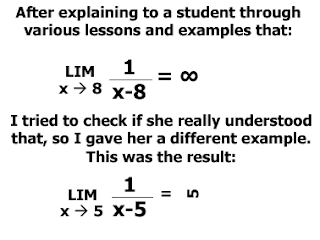Shecky, who blogs at Math Frolic had a link to a site with some math jokes... This was the one that gave me the best chuckle.

Addendum: My Apologies to Steven Colyer whose blog "Multiplication by Infinity" is the blog I linked to above. Sorry not to have credited Steven in the first place... just must have been a busy day. Mia Culpa

### Physics and Poker and NPR, Oh My!

The folks at "All Things Considered" are talking about how physics may make you a better poker player.

A few years ago, physicist Jeff Harvey invited Eduard Antonyan to a game of poker at a friend's house. Antonyan was a graduate student of Harvey's at the time, in the physics department at the University of Chicago.

"I invited Eduard to play because we're always looking for new victims," Harvey tells NPR's Guy Raz. "But it didn't exactly work out that well."

## Monday, 25 October 2010

### They Found a Way to Measure How Long it Takes to Fall in Love?

Falling in love can elicit not only the same euphoric feeling as using cocaine, but also affects intellectual areas of the brain. Falling in love only takes about a fifth of a second. The findings raise the question: "Does the heart fall in love, or the brain?"

Here is the link on Science Daily...see if you can figure out what they are measuring, or how they measured it... Keep in mind you have to put two people who are not in love in a situation in which they fall in love, and be measuring love (somehow) when it happens.

## Sunday, 24 October 2010

### More Harmonic Thoughts

I got a note after my last post that reminded me how little Julian Havel seems to be known in the US. Denise, of Let's Play Math, related that her local library had NO books by him. Impossible... No, I don't mean that it is impossible they have no books by him (it should be)...but that is one of his really good books.

Havel is (or was) a headmaster at a British College (sort of like the last year of our HS in the US).

In his book, Impossible, he has a chapter on the Harmonic Series in which he mentions a couple of more interesting notes I might have added for students.

For example, it is quite easy to see that H1= 1, and H2= 3/2. H6= 1 + 1/2 + 1/3 + 1/4 + 1/5 + 1/6 = 2 9/20... and those three are the only ones in the entire infinite series that end in a terminating decimal. Havel states that, "it can be proved" but does not... anyone know an tractable proof for that to approach Pre-calc students?

Another quick point to mention is the extremely slow rate at which the function diverges.... Ralph Boas Jr was co-finder of the smallest n for which Hn> 100. It turns out it takes more than 15 1042 terms to reach 100. Which means it is adding less than .01 each step... and yet... as Borovik cautions us... It Diverges..

## Friday, 22 October 2010

### A Nice Presentation of the Harmonic Series

Here is a nice video here about the Harmonic series. I would love for all my Pre-calc and calc students to see this video just to see the really nice proof by contradiction that the series is infinite. The paradox itself is just a bonus, and the fact that the squares add up to a value involving pi is a beautiful story that ought not to be popped out as an aside... sometimes we need to take the time to dig a little deeper into what Euler has given us... but a nice presentation all the same. Enjoy, but remember the adomonition that Borovik gave to his students,
" Today I said to the calculus students, "I know, you're looking at this series and you don't see what I'm warning you about. you look at it and you think, 'I trust this series. I would take candy from this series. I would get in a car with this series.' But I'm going to warn you, this series is out to get you. Always remember: The harmonic series diverges. Never forget it.

For more about the harmonic series, here and here and one more here, but surely there are more...use the search function.

### DaVinci Was Right

I subscribe to eGFI, a site that is there to encourage future students to go into Engineering. I recommend it to students who ask about or seem to want to go into Engineering. Today they had this on the site, with the video above..

Human-Powered Plane Flies Like A Bird

"For the past four years, Todd Reichert, an engineering student at the University of Toronto, has been working to perfect one of Leonardo Da Vinci’s greatest concepts – an ornithopter.

An ornithopter is a human-powered aircraft that flies by flapping its wings, and with the help of 30 other students, as well as \$200,000, Reichert made history by building such a vehicle and piloting a sustained flight.

Called the Snowbird, the aircraft is made of carbon fiber, foam and balsa wood, and weighs less than 93 pounds. Its wingspan is 104 feet, which is comparable to that of a Boeing 737. The vehicle works by pumping a set of pedals attached to pulleys and lines that bring down the wings in an elegant flapping motion.

Leonardo Da Vinci conceived the ornithopter back in 1485. For much of his life, Da Vinci was fascinated by the phenomenon of flight and produced many sketches of flying machines; however, he never actually made one."

### The Bead by Mamikon's Visual CaclulusOver the last few days I came upon a way to explain the volume of a bead with an (almost) non-calculus approach. My solution was inspired by a comment posted by Arjen Dijksman. I admitted I didn't understand his suggestion to use Mamikon's visual calculus, so he did what good teachers do. He posted a very clear explanation that even I could not miss. It's a beautiful blog, pretty math... check it out..

## Thursday, 21 October 2010

### A Non-calculus Explanation for the Volume of a BeadA few days ago I wrote about the "surprising constant" that a bead formed by drilling a hole through the center of a sphere had a volume that was dependent only on the height of the remaining bead. At the time I admitted I could not think of a good "non-calculus" explanation for why the volume for a given bead height was a constant.

Afterward Arjen Dijksman,(see his blog) who regularly provides helpful insights, suggested that the volume could be explained using the ideas of Mamikon Mnatsakanian that I discussed here. While I was not able to see Arjen's solution, it did lead me to realize that a "non-calculus" proof existed (with some allowances).

Thinking of Mamikon's annular sweep led me to realize that every cross-section through the bead perpendicular to the axis of the drilled hole would be an annulus. In fact, using R as the original sphere's radius, r as the radius of the hole, and h as the distance above the center of the sphere where the cross section was taken, the area of the annulus would be Pi times (R2 - r2-h2), which is just the area of a circle.

Now by Cavalieri's Principal, "If, in two solids of equal altitude, the sections made by planes parallel to and at the same distance from their respective bases are always equal, then the volumes of the two solids are equal."

Several candidates exist with circular cross-sections; cylinders, cones, and spheres are examples. The cross-sections of the bead went from zero at the top, to a maximum at the center, so the cylinder is excluded. We can exclude the cone since their radii decrease linearly from top to bottom and our bead does not. And since we are already tempted with the idea that the volume of the bead is the same as a bead with a radius equal to half the total height of the bead, why not try to compare those?

To make the notation easier, I decided to call the height of the bead 2H (letting H2 equal the value (R2 - r2). So the cross sectional area of the sphere with raidus H at any height, h, above the center will be Pi(H2 - h2). As we have shown above, the cross-sectional area of the bead at the same height h is Pi (R2 - r2-h 2). and since H2 is equal to (R2 - r2) these two are equal.

So we have proven that the volume of the bead is the same as the volume of a sphere with the same height;... but did we use calculus? I fear that the proof of Cavalieri's Principal is founded in the calculus, and thus we still have a "calculus-based" explanation...but it's the best I have to offer to date.

## Tuesday, 19 October 2010

### Folium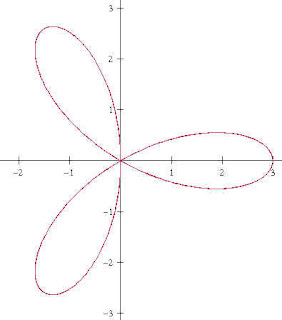I realized in Caculus class the other day that I didn't have a link for folium on my Mathwords webpage, so I decided to write one here and just put a link...
--------------------------------------------
Folium is the Latin word for leaf. Student’s frequently are introduced to them in learning to graph polar curves such as r=sin(3*theta) which is a trifoil, or three leaf, sometimes called a “rose” (shown above).

The one that came up in my Calculus class a few days ago was one of the more widely known mathematical folia, the “Folium of Des Cartes”, x3 + y3=3xy. A nice chance to use the newfound skill of implicit differentiation. This curve was created by Des Cartes to challenge the methods of Fermat in finding extrema in the early days of the emerging calculus. I should mention that both men found the extrema without the calculus of limits as we now know it. I mentioned Des Cartes method, as extended by Hudde, in an earlier posting here.The Folium can be rendered in parametric form by using the substitution y=tx to give

x3 + (tx)3 = 3tx2 and dividing out x2 we get to

x + t3 x = 3t... This can be factored for x to get x(1+t3)= 3t.... or

x= (3t) / (1+t3) and thus by y=xt we get y= (3t2)/ (1+t3)

I recently came across this neat stamp image with a picture of Des Cartes and his Folium.